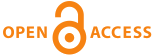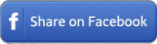Home|Journals|Articles by Year|Audio Abstracts RSS - TOCin any field in Title in Summary in Keywords in Authors in journals

 Original ResearchMed-Science. 2018; 7(2): 350-4

#### Estimation of total prostate specific antigen values through artificial intelligence modelling

Seyma Yasar, Ali Beytur, Cemil Colak.

##### Abstract

It has been indicated that total prostate specific antigen (PSA) screening, one of the serum markers used for the diagnosis of prostate cancer, has been clinically beneficial. In this research, it was aimed to estimate the total PSA values by Multilayer Perceptron (MLP) artificial neural network (ANN) model. Data on total PSA values in this study (n = 1422) were randomly selected using the structured query language (SQL) from the database of patients records of Urology Department of Medical School at Inonu University. Total PSA values as a target/dependent variable, and age (year), blood group (A/B/0/AB), Exitus (EX) status (alive/death), Lymphocyte (LY) (%), Hemoglobin (HGB) (g / dL), Neutrophil (NE) (%), Albumin (g / dL), Calcium (mg / dL), Mean Corpuscular Hemoglobin (MCH), Leukocyte count (WBC) (103 / ml), Platelet (PLT) (103/ ml) as predictor variables were evaluated in the analyses. Outlier/extreme observations were analysed, and quantitative variables were rescaled by the transformation of Z-score or Box-Cox, and the MLP ANN model was constructed to estimate the total PSA values after variable selection method was used. Estimation performance of the model was examined by the values of mean absolute error, standard deviation and correlation coefficient. The MLP ANN model was created using a total of 1422 data sets as 993 of which were in training and 429 in the testing. Values of the mean absolute error, standard deviation, and correlation coefficient were calculated for training data set as; 0.744, 0.895 and 0.452; for the test data set as; 0.773, 0.935 and 0.355. The estimated accuracy of the generated model is predicted as 20.3%. In the MLP ANN model, the importance levels of the variables were obtained as 0.33 for HGB, 0.22 for NE, 0.16 for Calcium, 0.13 for PLT, 0.10 for age and 0.06 for EX. The MLP ANN model was established for the estimation of the total PSA values based on the selected variables, and calculated the importance levels of the related variables. Better prediction results in the estimation of total PSA values can be provided by using different additional variables, various resampling methods and alternative models.

Key words: Artificial intelligence modelling, risk factors, total prostate specific antigen

 Share this ArticleOnline Article Submission
• ejmanager.com
• ojshosting.net